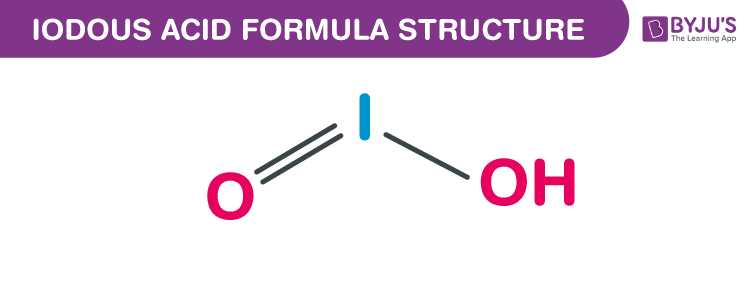# Iodous acid Formula

Iodous acid formula, also named as Hypoiodic acid formula or Iodic acid formula is discussed in this article. Hypoiodic acid is a conjugate acid of an iodite and an iodine oxoacid. The molecular or chemical formula of Iodous acid is HIO2.

The salts of this chemical compound are named iodites. These are extremely unstable, observed but never isolated. They disproportionate quickly to molecular iodates and iodine. Its molecular formula is 159.911 g/mol.

## Iodous acid Formula Structure## Properties Of Iodous acid Formula

 Chemical formula HIO2 Molecular weight 159.911 g/mol Hydrogen bond donor 1 Conjugate base Iodite Complexity 10.3

The number of hydrogen bond donor is one whereas the number of hydrogen bond acceptors are two. The monoisotopic mass is equal to 159.902 g/mol.